The equivalent resistance across AB is :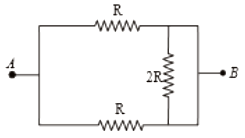1.  2R

2.  R/2

3.  4R/3

4.  3R/2

Subtopic:  Combination of Resistors |
To view explanation, please enrol in the course below.
NEET 2021 - Achiever Batch - Aryan Raj Singh

Difficulty Level:

The equivalent resistance between points A and B in the circuit shown in the figure is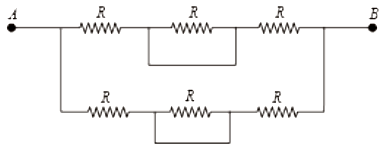1.  6R

2.  4R

3.  2R

4.  R

Subtopic:  Combination of Resistors |
To view explanation, please enrol in the course below.
NEET 2021 - Achiever Batch - Aryan Raj Singh

Difficulty Level:

The drift velocity of the electrons in a current-carrying  metallic conductor is of the order of

1.

2.

3.

4.  ${10}^{-4}\mathrm{m}/\mathrm{s}$

Subtopic:  Current & Current Density |
To view explanation, please enrol in the course below.
NEET 2021 - Achiever Batch - Aryan Raj Singh

Difficulty Level:

In the circuit shown in the figure, the effective resistance between A and B is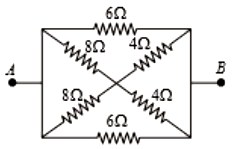1.  2$\mathrm{\Omega }$

2.  4$\mathrm{\Omega }$

3.  6$\mathrm{\Omega }$

4.  8$\mathrm{\Omega }$

Subtopic:  Combination of Resistors |
To view explanation, please enrol in the course below.
NEET 2021 - Achiever Batch - Aryan Raj Singh

Difficulty Level:

For the circuit shown in the figure, the value of R must be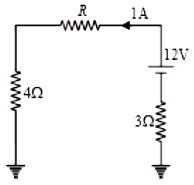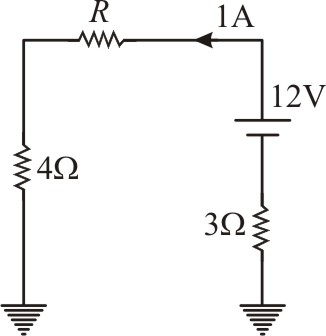1.  3$\mathrm{\Omega }$

2.  4$\mathrm{\Omega }$

3.  5$\mathrm{\Omega }$

4.  6$\mathrm{\Omega }$

Subtopic:  Kirchoff's Voltage Law |
To view explanation, please enrol in the course below.
NEET 2021 - Achiever Batch - Aryan Raj Singh

Difficulty Level:

If I be the current limit of a fuse wire of length l and radius r, then select the appropriate relation

(1)

(2)

(3)

(4)

Subtopic:  Heating Effects of Current |
To view explanation, please enrol in the course below.
NEET 2021 - Achiever Batch - Aryan Raj Singh

Difficulty Level:

The resistance of platinum wire at 0$°\mathrm{C}$ is 22.05 $\mathrm{\Omega }$ and at 100$°\mathrm{C}$ it becomes 22.70 $\mathrm{\Omega }$. When the wire is heated to a temperature of t$°\mathrm{C}$ the resistance of wire becomes 24.91$\mathrm{\Omega }$. The value of t is

1.  220

2.  440

3.  310

4.  550

Subtopic:  Derivation of Ohm's Law |
To view explanation, please enrol in the course below.
NEET 2021 - Achiever Batch - Aryan Raj Singh

Difficulty Level:

Two wire A and B of copper have equal masses and the lengths are 10 cm and 20 cm respectively. If resistance of A be RA and B be RB, then the value of $\frac{{R}_{A}}{{R}_{B}}$ is

1.

2.

3.

4.   $4$

Subtopic:  Derivation of Ohm's Law |
To view explanation, please enrol in the course below.
NEET 2021 - Achiever Batch - Aryan Raj Singh

Difficulty Level:

The sensitivity of a potentiometer can be increased by

1.  increasing the potential gradient

2.  decreasing the length of potentiometer wire

3.  decreasing the current in the potentiometer wire

4.  all of these

Subtopic:  Meter Bridge & Potentiometer |
To view explanation, please enrol in the course below.
NEET 2021 - Achiever Batch - Aryan Raj Singh

Difficulty Level: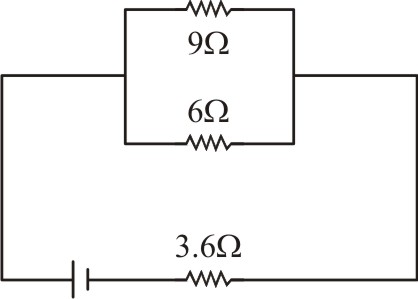1.  36V

2.  18V

3.  9V

4.  Zero

Subtopic:  Heating Effects of Current |
To view explanation, please enrol in the course below.
NEET 2021 - Achiever Batch - Aryan Raj Singh

Difficulty Level: